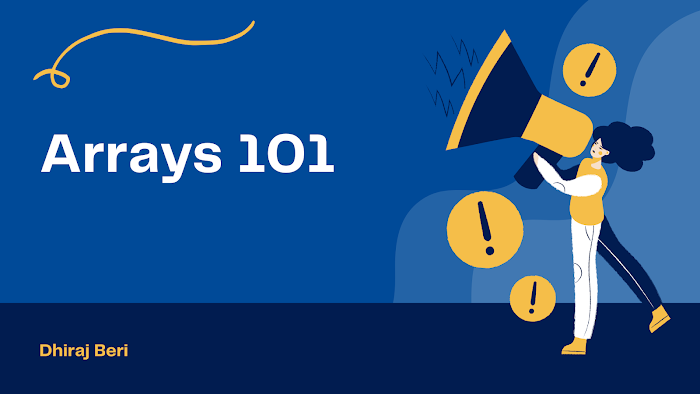# Finding duplicates in an array

Condition 1: If Array is sorted.

`#include <stdio.h>int main(){    int arr[] = {3, 6, 8, 8, 10, 12, 15, 15, 15, 20};    int lastDuplicate = 0;    int n;    for (int i = 0; i < n; i++)    {        if (arr[i] == arr[i + 1] && arr[i] != lastDuplicate)        {            printf("%d\n", arr[i]);            lastDuplicate = arr[i];        }    }    return 0;}`

Count duplicates in a sorted array

`#include <stdio.h>int main(){    int arr = {3, 6, 8, 8, 10, 12, 15, 15, 15, 20};    int lastDuplicate = 0;    int n, j;    for (int i = 0; i < n - 1; i++)    {        if (arr[i] == arr[i + 1])        {            j = i + 1;            while (arr[j] == arr[i])            {                j++;            }            printf("%d is appearing %d times\n", arr[i], j - i);            i = j - 1;        }    }    return 0;}`

Using Hash Table (Best) - works for sorted and unsorted arrays

`#include <stdio.h>int main(){    // int arr = {3, 6, 8, 8, 10, 12, 15, 15, 15, 20};    int arr = {8, 3, 6, 4, 6, 5, 6, 8, 2, 7};    int h[] = {0, 0, 0, 0, 0, 0, 0, 0, 0, 0, 0, 0, 0, 0, 0, 0, 0, 0, 0, 0, 0};    int n = 10, i;    for (i = 0; i < n; i++)    {        h[arr[i]]++;    }    for (i = 0; i < 20; i++)    {        if (h[i] > 1)        {            printf("%d is appearing %d times\n", i, h[i]);        }    }    return 0;}`

Finding duplicates in the unsorted array

`#include <stdio.h>int main(){    int a[] = {8, 3, 6, 4, 6, 5, 6, 8, 2, 7};    int n = 10;    int count;    for (int i = 0; i < n - 1; i++)    {        count = 1;        if (a[i] != -1)        {            for (int j = i + 1; j < n; j++)            {                if (a[i] == a[j])                {                    count++;                    a[j] = -1;                }            }            if (count > 1)                printf("%d %d\n", a[i], count);        }    }    return 0;}`